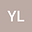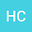loading page

Bifurcation and comparison of a discrete-time Hindmarsh-Rose model
••Hongjun Cao
Beijing Jiaotong University
Author Profile## Abstract

In this paper, a discrete-time Hindmarsh-Rose model is obtained by a nonstandard finite difference (NSFD) scheme. Bifurcation behaviors between the model obtained by the forward Euler scheme and the model obtained by the NSFD scheme are compared. Through analytical and numerical comparisons, much more bifurcations and dynamical behaviors can be obtained and preserved by using the NSFD scheme, in which the integral step size can be chosen larger relatively due to its better stability and convergence than those in the forward Euler scheme. It means that the discretetime model obtained by the NSFD scheme is closer to the original continuous system than the discrete-time model obtained by the forward Euler scheme. These confirmed results can at least guarantee true available numerical results to investigate complex neuron dynamical systems.